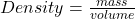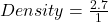## ASAPP PLS HELP MEE The density of aluminum is 2.7 g/cm^3. Which of the following statement is true? A) 1 g of aluminium has

Question

ASAPP PLS HELP MEE
The density of aluminum is 2.7 g/cm^3. Which of the following statement is true?

A) 1 g of aluminium has a volume of 1 cm^3

B) 2.7 g of aluminium has a volume of 1 cm^3

C) 1 g of aluminium has a volume of 2.7 cm^3

D) 2 g of aluminium has a volume of 7 cm^3​

in progress 0
7 months 2021-07-17T08:36:34+00:00 1 Answers 6 views 0

B) 2.7 g of aluminium has a volume of 1 cm^3

Explanation:

Density can be defined as mass all over the volume of an object.

Simply stated, density is mass per unit volume of an object.

Mathematically, density is given by the equation;If the density of aluminum is 2.7 g/cm³, it simply means that 2.7 g of aluminium has a volume of 1 cm³

Check:

Given the following data;

Mass = 2.7 grams

Volume = 1 cm³

Substituting into the formula, we have;Density = 2.7 g/cm³